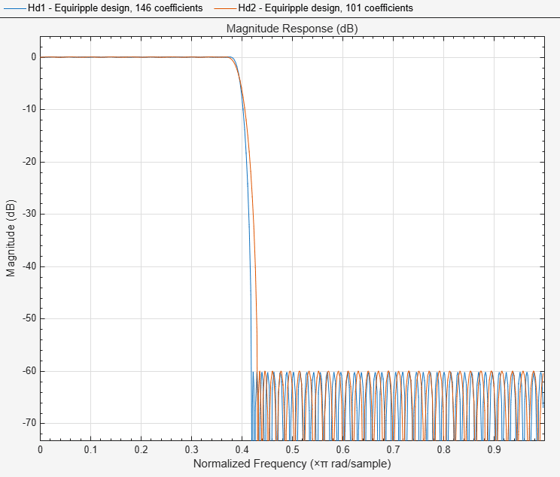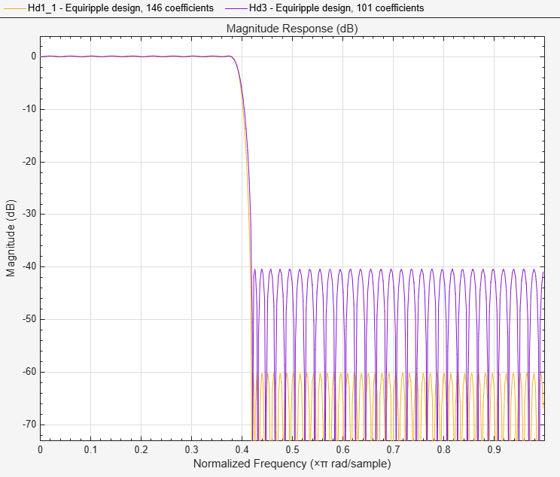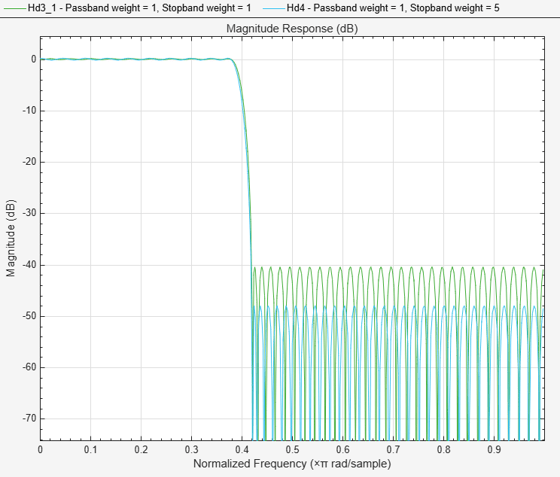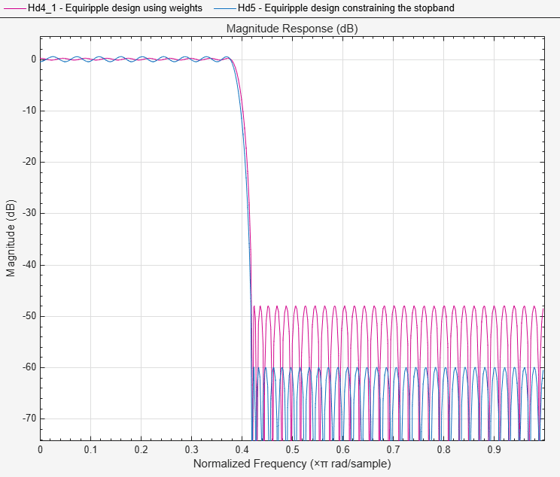# Controlling Design Specifications in Lowpass FIR Design

This example shows how to control the filter order, passband ripple, stopband attenuation, and transition region width of a lowpass FIR filter.

### Controlling the Filter Order and Passband Ripples and Stopband Attenuation

When targeting custom hardware, it is common to find cases where the number of coefficients is constrained to a set number. In these cases, minimum order designs are not useful because there is no control over the resulting filter order. As an example, suppose that only 101 coefficients could be used and the passband ripple/stopband attenuation specifications need to be met. We can still use equiripple designs for these specifications. However, we lose control over the transition width which will increase. This is the price to pay for reducing the order while maintaining the passband ripple/stopband attenuation specifications.

Consider a simple design of a lowpass filter with a cutoff frequency of 0.4*pi radians per sample:

``` Ap = 0.06; Ast = 60; Fp = 0.38; Fst = 0.42; Hf=fdesign.lowpass('Fp,Fst,Ap,Ast',Fp,Fst,Ap,Ast);```

Design an equiripple filter:

` Hd1 = design(Hf,'equiripple','systemobject',true);`

Set the number of coefficients to 101, which means setting the order to 100:

``` N = 100; Fc = 0.4; setspecs(Hf,'N,Fc,Ap,Ast',N,Fc,Ap,Ast);```

Design a second equiripple filter with the given constraint:

` Hd2 = design(Hf,'equiripple','systemobject',true);`

Measure the filter variables of the second equiripple filter, and compare the graphs of the first and second filters:

` measure(Hd2)`
```ans = Sample Rate : N/A (normalized frequency) Passband Edge : 0.37316 3-dB Point : 0.39285 6-dB Point : 0.4 Stopband Edge : 0.43134 Passband Ripple : 0.06 dB Stopband Atten. : 60 dB Transition Width : 0.058177 ```
``` hfvt = fvtool(Hd1,Hd2,'Color','White'); legend(hfvt,'Equiripple design, 146 coefficients', ... 'Equiripple design, 101 coefficients')```The transition has increased by almost 50%. This is not surprising given the almost 50% difference between 101 coefficients and 146 coefficients.

### Controlling the Transition Region Width

Another option when the number of coefficients is set is to maintain the transition width at the expense of control over the passband ripple/stopband attenuation.

``` setspecs(Hf,'N,Fp,Fst',N,Fp,Fst); Hd3 = design(Hf,'equiripple','systemobject',true); measure(Hd3)```
```ans = Sample Rate : N/A (normalized frequency) Passband Edge : 0.38 3-dB Point : 0.39407 6-dB Point : 0.4 Stopband Edge : 0.42 Passband Ripple : 0.1651 dB Stopband Atten. : 40.4369 dB Transition Width : 0.04 ```
``` hfvt2 = fvtool(Hd1,Hd3,'Color','White'); legend(hfvt2,'Equiripple design, 146 coefficients',... 'Equiripple design, 101 coefficients')```The differences between using 146 coefficients and using 101 coefficients is reflected in a larger passband ripple and a smaller stopband attenuation.

It is possible to increase the attenuation in the stopband while keeping the same filter order and transition width by the use of weights. Weights are a way of specifying the relative importance of the passband ripple versus the stopband attenuation. By default, passband and stopband are equally weighted (a weight of one is assigned to each). If we increase the stopband weight, we can increase the stopband attenuation at the expense of increasing the stopband ripple as well.

``` Hd4 = design(Hf,'equiripple','Wstop',5,'systemobject',true); measure(Hd4)```
```ans = Sample Rate : N/A (normalized frequency) Passband Edge : 0.38 3-dB Point : 0.39143 6-dB Point : 0.39722 Stopband Edge : 0.42 Passband Ripple : 0.34529 dB Stopband Atten. : 48.0068 dB Transition Width : 0.04 ```
``` hfvt3 = fvtool(Hd3,Hd4,'Color','White'); legend(hfvt3,'Passband weight = 1, Stopband weight = 1',... 'Passband weight = 1, Stopband weight = 5')```Another possibility is to specify the exact stopband attenuation desired and lose control over the passband ripple. This is a powerful and very desirable specification. One has control over most parameters of interest.

``` setspecs(Hf,'N,Fp,Fst,Ast',N,Fp,Fst,Ast); Hd5 = design(Hf,'equiripple','systemobject',true); hfvt4 = fvtool(Hd4,Hd5,'Color','White'); legend(hfvt4,'Equiripple design using weights',... 'Equiripple design constraining the stopband')```